# Bridge to Mathematics

## Living Math

Mathematics can tend to be a topic that bewilders people or leaves them cold.  It can be regarded as a complex subject that people suffered through in school and are glad to leave behind.  Where it arises this is a most unfortunate circumstance as mathematics lies at the very core of creation and articulates much of the structure that emerges through creation.

Our difficulty in relating to math can arise from the way it is formulated and taught.  The elements of math, number, geometric shapes, etc., tend to be regarded as static, detached entities residing in cold cerebral isolation.  We relate to and manipulate them with relentless logic.  Yet there is an awakening awareness of a deeper connection an almost mystical significance bubbling up from the depths of mathematics.  Despite the pervasive logical rationalism there is a beauty, an art, a sense of meaning and value that shines through in the magnificence of the structures.

We can come to know number, the elements of geometry, etc. as living realities.  They carry a logic in action that helps shape creation.  But they also carry connection, a subtle and powerful connection that brings many facets of creation into relationship.  Exploring such connection is of great value in rebuilding relationship with the subtle levels both in creation and in our own being.

The topic of sacred geometry attracts considerable interest.  However this can still be somewhat static and detached.  We can relate to our friends in the realm of number in more real terms as living entities in terms of a living geometry or living math.  We can engage conversation and find that they have much to reveal to us.  This is an altogether more satisfying and interesting relationship.  Let us follow the chains of connection and see where they might lead.

## Krystal Spiral

The Krystal Spiral emerges through the progressive rotation and expansion of the Kathara Grid.  Although simple in concept this process carries a powerful mathematical structure derived from the properties of the Kathara Grid.

Every rotation through 90°, e.g. from centers 6 to 12 in the diagram over, yields an expansion by a factor of 2.  This leads to the following number sequence:

1, 2, 4, 8, 16, 32, 64, 128, 256, ….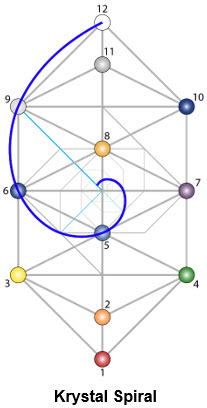## Pascal Number Triangle

There is progressive multiplication by 2 in this sequence.  Now 2 comprises two elements or units, i.e. 1 + 1.  If instead of merging these two numbers in 2 we preserve their separate identity in the multiplication process we get the triangular number pattern illustrated below.  Instead of multiplying the 2’s directly we multiply the component (1 + 1)’s.  This process illustrates the multiplication and preservation of diversity.  Instead of merging numbers it preserves their separate identity and allows them express diversity and new levels of structure.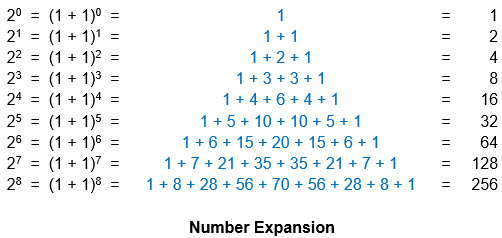The derivation can be explained briefly by example as follows.  (a + b)2  =  a2 + 2a.b + b2.  Replace ‘a’ & ‘b’ by 1’s and we get (1 + 1)2  = 1 + 2 + 1.  This corresponds to the third row in the list above.  A similar process applies to all the other rows.  The triangular number pattern shown in blue in the center of the list builds up progressively.

The numbers can be assembled relatively easily as follows.  Each number row in the triangle is obtained by multiplying the previous row by (1 + 1).  For example take the fourth row (1 + 3 + 3 + 1) and multiply by (1 + 1) to get the fifth row as follows:

(1 + 3 + 3 + 1) x (1 + 1)   =           1 + 3 + 3 + 1 , repeat row below and shift a position                                                                     1 + 3 + 3 + 1       , add two rows vertically                                                                                    =     1 + 4 + 6 + 4 + 1 , to get next row.

This procedure can be repeated down through the triangle to generate as many rows as desired.  Another procedure that can be used to generate the Pascal numbers is to note that every number is the sum of the two numbers left and right in the row above.  For example in the last row in the diagram ‘8’ = ’1’ + ‘7’ from the row above, ‘28’ = ‘7’ + ‘21’, etc.  We then simply pad out the wings with ‘1’s.

This number pattern is well known in mathematics as the Pascal Triangle.  It is named after the famous seventeenth century French mathematician and philosopher Blaise Pascal, who developed it.  It plays a significant role in mathematics.  Here the numbers are known as binomial coefficients and have important applications in algebra, statistics, etc.  The term binomial relates to 2 elements, reflecting the significance of 2 in their structure.

This Pascal number pattern carries a lot of structure within it.  Traditionally even numbers are regarded as female and odd as male.  If we apply this gender polarity to the Pascal number triangle a pattern emerges.  The even / female numbers are color coded with yellow background in the Pascal Triangle over.  The odd / male numbers are in blue text and white background.  We see a pattern of inner triangles building up within the structure.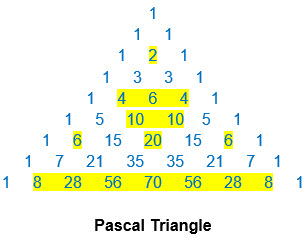This pattern is continued to a greater level of detail in the gender coded Pascal Triangle in the diagram below.  Every even number position is tiled yellow and odd number positions blue.  This illustrates the triangular pattern more clearly.

Looking at the pattern from the top down, we have one blue tile in the first / topmost row.  This combines with two further blue tiles in the second row to form a blue / male triangle.  In the next two rows below it this triangle is repeated twice to make a collection of 3 such triangles in the first 4 rows with a yellow / female tile in the center.  This whole unit is then repeated twice in the next 4 rows with a corresponding yellow / female triangle in the center pointing downwards.  Again this pattern is repeated twice in the next 8 rows below it with a yellow downward pointing triangle in the center.  This progression continues indefinitely building ever greater detail.

If we count the number of blue tiles in the row at the base of each triangular unit we get the number progression – 1, 2, 4, 8, 16, … , corresponding to the first, second, fourth, eighth, etc. rows.  This corresponds to the Krystal Spiral number sequence.

Also if we count the total number of blue tiles in each triangular unit we get – 1, 3, 9, 27, 81, …  This corresponds to the Merkaba Spiral expansion sequence based on powers of 3.  So both the Krystal and Merkaba Spiral number sequences are embedded in the Pascal Triangle.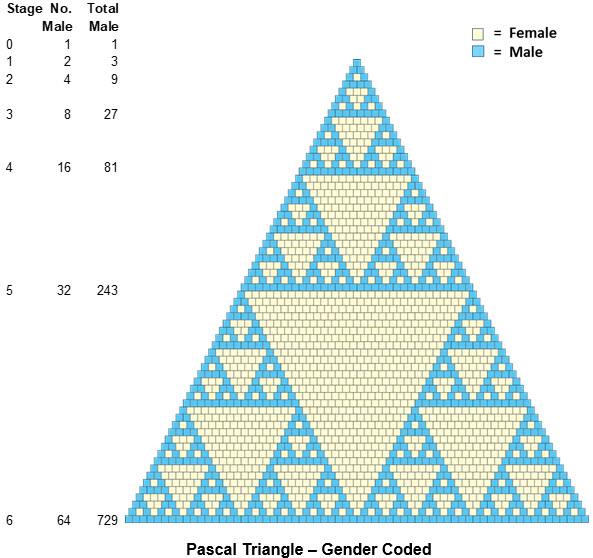We can smooth out the tiles to illustrate the triangular pattern more clearly in the diagram below.  This yields a structure known as the Sierpinski Triangle.  It is named after the Polish mathematician Waclaw Sierpinski, who described it in 1915.

Here we can see how the pattern of triangles builds up.  We can see it expanding from the top point downwards.  We also see it forming from the outside in.  Take the sequence of triangles in the Triangle Formation diagram further down.  Here we take a blue triangle and embed a smaller replica in yellow with dimensions reduced by half and inverted in the middle of it.  This divides the original triangle into 4 smaller triangles.  Three of these in blue are pointing upwards while the middle yellow one points downwards.

Now take each of the smaller blue triangles and repeat the process with these, i.e. place a still smaller yellow triangle in the center of each of these.  This is represented in the third pattern in the Triangle Formation sequence.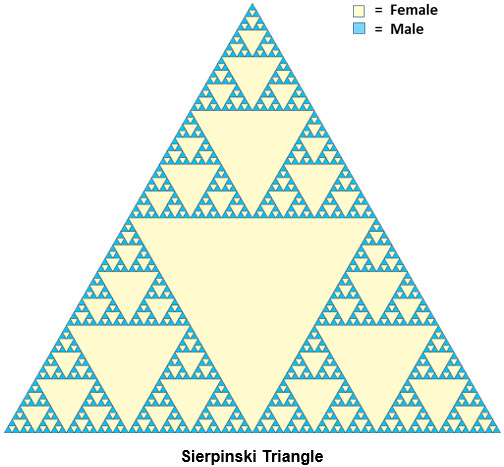This process can be continued indefinitely.  Every upward pointing blue triangle is subdivided in progressively greater detail.  This generates the pattern we see in the Sierpinski Triangle above.

This manner of subdividing a triangle generates a tetrahedron, as illustrated in the Tetrahedron Formation diagram below.  All we have to do is take one of these triangles with a yellow center as in the middle of the Triangle Formation sequence below.  Then fold the blue triangles upwards until their outer points meet at an apex and we have a tetrahedron.  The triangle divided in 4 smaller triangles is a 2D representation of a tetrahedron.

The tetrahedron is the basic element of Merkaba structure.  This triangular pattern derived from the Pascal number triangle in fact encodes all the key elements of Merkaba mechanics.  It illustrates the process of tetrahedron formation.  All the electrical / male triangles are pointing up wards and the magnetic / female ones point downwards.  It captures the polarity alignment of the electrical / magnetic Merkaba spirals.  In the basic fourfold division of the triangles there is a 3:1 ratio between the electrical and magnetic elements.  This is a key component of the balance of electrical to magnetic Merkaba spiral spin speeds.

This is an excellent example of encryption in action.  One would normally expect such a number pattern to be structure less or random.  Instead we have a fairly simple process revealing very specific structure with ever greater degrees of sophistication.  Intelligence and consciousness emerge through the process to express structure in unexpected ways.  Structure shapes creation.  But encryption or embedded intelligence / consciousness in turn shape structure and condition what flows into creation through it.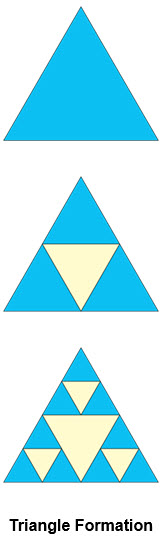t is particularly interesting to see the Krystal Spiral feeding into this number pattern and to see the key elements of Merkaba structure emerge from it.  The number pattern also embeds both the Krystal and Merkaba Spiral number sequences as we have seen above.This structure is a hologram – the pattern of the whole is present at every level down to the finest detail.  We can look at any level and see the same pattern repeated.  It is also an excellent example of a fractal structure.  It is a self similar pattern with infinitely many levels of detail.

## Diversity

Diversity is important in creation.  It is what creates variety, interest and excitement.  Imagine if the universe were all male or female!  It is the difference that makes creation interesting and allows for new creative possibilities.  In the number expansion in the Krystal Spiral sequence treating the ones that make up 2 as separate allows for diversity to emerge.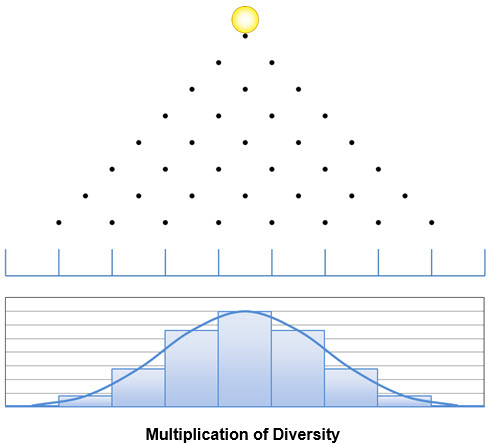Expansion of diversity follows a pattern described by the numbers in the Pascal triangle.  We can take a simple and frequently used example to illustrate the process.  Consider a coin falling down through a coin chute in an amusement arcade, as illustrated in the diagram above.  It has two options, falling right or left, at every peg.  The coins are collected in bins at the bottom.  There is randomness at each collision point and this propagates down through the grid.  Nonetheless there is a pattern to the way the coins collect at the bottom.  This pattern becomes progressively more apparent as we run more coins through the grid.  It is illustrated by the graph below the grid.  With a finite number of bins the graph is stepped.  As we add levels to the grid and correspondingly more bins at the bottom it becomes progressively smoother.

The distribution of coins at the bottom is represented by the numbers in the Pascal triangle above.  We can take any level of the grid and the relative frequency of coins at that level will be represented by the numbers in the corresponding level of the Pascal triangle.  For example with 3 pegs and 4 bins the frequency of coins falling into the bins would converge towards the proportion 1, 3, 3, 1, as in that level of the Pascal triangle.

The underlying process is general whether dealing with a coin chute, propagation of characteristics through parents, generations, etc.  There is propagation of diversity.  This process leads to a natural clustering around average values but with a spread also, e.g. average height for people, life expectancy, etc.  With a finite number of steps as in the coin chute above the process is described by a mathematical structure known as the ‘binomial distribution’.  This contains both the numbers from the Pascal triangle and inverted powers of 2.

As the number of steps gets very large and the distribution smoother the binomial distribution above becomes increasingly unwieldy.  It transitions into another very important mathematical structure known as the normal distribution.  This is the famous ‘Bell Curve’.  It is used widely to describe clustering processes around a mean value, e.g. intelligence levels, failure rates of equipment, voter choices in an election, etc.

When dealing with a finite number of discrete steps the clustering pattern is described by powers of 2.  As we move to a large number of steps and an increasingly smooth spread we transition to powers of ‘e’ instead of 2 to describe this.  e = 2.718 … , known as the exponential value, is a very important number that lies at the heart of mathematics.  We will meet it again shortly.  The general shape of the Bell Curve is shown in the graph below.  This brings together two of the most important numbers in mathematics – ‘e’ = 2.718 … and π = 3.142 … .  The exact mathematical description of the Bell Curve is a bit more complex that this, but the diagram illustrates the key elements.

This process by which diversity propagates emerges from and is governed by the Krystal Spiral.  The Krystal Spiral generates a number sequence, from which the Pascal number triangle emerges.  This in turn conditions key clustering patterns for diversity in creation.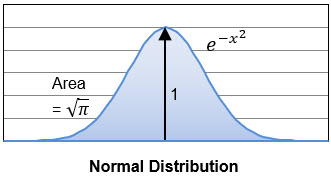This builds important connection between the Krystal Spiral and some core mathematical structures.  It bridges between spirit and number to help build sacred geometry and math.

## Exponential Growth

The Krystal Spiral yields a process of natural growth.  This is evident in the expansion out from the center as the spiral rotates around this center.  It is most clearly illustrated in the spiral number sequence at the 90° points of rotation, i.e. 1, 2, 4, 8, 16, 32, 64, …  Here we have a natural doubling at every quadrant stage of expansion, as illustrated in the diagram over.  This expansion by repeated multiplication is known as exponential growth.
The Krystal Spiral can also contract inwards in a spiral that approaches zero.  We see this contraction by moving leftwards in the diagrams from stage 0 towards negative stage numbers.  This process is illustrated in the second diagram over.  It is simply the expansion process going backwards, i.e. it is the same process going in the opposite direction to expansion.
In this case the numbers reduce by 2 with every stage of contraction.  They go from 1 to ½, ¼, 1/8, 1/16, 1/32, 1/64, …  We can also regard these stages as inner growth from very small / fractional numbers upwards towards 1.

Now the sum of all the inner stages ½ + ¼ + 1/8 + 1/16 + 1/32 + 1/64 +, …  equals the base stage 1.

Likewise every stage of Krystal Spiral expansion equals the sum of all the prior stages, including the inner stages approaching zero.  For example:

64 = 32 + 16 + 8 + 4 + 2 + 1 + ½ + ¼ + 1/8 + 1/16 + 1/32 + 1/64 +, …

Here we are dealing with a growth process that increases / decreases in pronounced steps by a factor of 2.  There are very discrete jumps from one stage to the next.  We don’t normally see such pronounced step changes from one stage to the next.

If we take money invested to earn interest it will grow over time.  For example it may take 10 years to double in value.  The process above would correspond to interest being applied to the account only every 10 years when the value doubles.  This is stepped growth with a doubling at every step, as illustrated in the first diagram over.  We have the original amount represented by 1.  After 10 years this increases by another 1 so that we get:

1 + 1 = 2
In practice we would expect to get interest on our money at much more frequent intervals than that.  We would expect interest to be applied at least yearly, as illustrated in the second diagram over.  In this case doubling over say 10 years would correspond to an increase of 1/10 or 10% per annum.  So after a year we have the original amount 1 plus the increase of 1/10, i.e. we get:

1 + 1/10 = 1.1

Now this annual interest or growth is compounded from year to year, i.e. the interest each year itself earns interest in subsequent years.  So after 10 years we get 1 + 1/10 multiplied by itself 10 times, i.e.:

(1 + 1/10)10 = 2.594 …

Applying interest growth yearly obviously is more beneficial than waiting for 10 years.  If we were to apply interest more frequently than this would there be any additional benefit?  What would the situation be if we were to get interest applied monthly?  In this case the doubling would be distributed and compounded over 10 x 12 = 120 steps instead of 10.  We simply replace 10 by 120 in the expression above to get:

(1 + 1/120)120 = 2.707 …

How about daily?  Here we have 10 x 365 = 3,650 steps to give:

(1 + 1/3,650)3,650 = 2.718 …

In the more general case of N steps we have:

(1 + 1/N)N

As N gets very large especially infinitely large this expression simplifies to:
Thus the benefit of applying interest or compounding growth more frequently tops out with a base gain over the period of 2.718 …

Now 2.718 … is the exponential number ‘e’ we have already encountered.  The expression above is the standard mathematical definition of ‘e’.  So as we move from stepped towards smooth growth the base that defines the growth transitions from 2 to ‘e’ = 2.718 … , i.e. the original 1 + 1 = 2 becomes (1 + 1/N)N = 2.718 …  Whereas powers of 2 are associated with discrete stepped growth, powers of ‘e’ serve exactly the same role for smooth continuous growth.

If we don’t compound the growth but wait for a step increase at the natural doubling period we get an increase by 2.  If we compound the growth continuously we will get an increase by 2.718 … over this same period.  The same 2.718 … growth will apply to the next such period.  So the base for continuous growth becomes 2.718 … instead of 2. This provides the standard definition for exponential growth, i.e. ex.

We have used the example of growth in money invested above to illustrate the principle.  However the process is universal.  It applies equally to any natural unconstrained growth, e.g. population growth, growth of a tree, economic growth, etc.  The time period for doubling isn’t critical.  We used 10 years in the example above for convenience.

Most growth tends to be fairly smooth.  Population growth for example does occur in terms of steps of one person at a time.  However that increment of one person in relation to a total population of millions for a country or around 7 billion for the planet is so small that the steps are smoothed out.  Likewise the growth of a tree or plant is more smooth than stepped.

This exponential growth process can be used equally to describe contraction or decay processes.  We simply apply negative index numbers instead of the positive ones for expansion / growth.  These decay processes apply for example to the cooling of a cup of coffee, the decay of a musical note or any sound, radioactive decay, etc.

We have seen above in the case of powers of 2 that all the inner stages before 1 (Stage 0) add up to 1, i.e. 1 = ½, ¼, 1/8, 1/16, 1/32, 1/64, …  The corresponding stages for the exponential function based on ‘e’ are illustrated in the diagram over.  Here Stage 0 has a value of 1.  All the stages prior to this sum to 1, i.e. the total area to the left of Stage 0 is 1.
Exponential growth beyond the value of 1 or Stage 0 is illustrated in the second diagram over.  The shape of the curve is the same as above but the scale is different.  With a smooth curve the base ‘e’ can be raised to any power ‘a’, i.e. ‘a’ can be any number along the X axis.  For a position ‘a’ the corresponding value is ea as shown.
We have seen above that each stage of powers of 2 expansion equals the sum of all the prior stages including the fractional stages approaching zero.  Likewise here the sum of all the values prior to ‘a’ sum to the value at ‘a’, i.e. ea as shown.  Thus the value at any point ‘a’ equals all the area to the left including that in the negative region beyond zero.  Each point integrates all the prior history.  In purely mathematical terms this property is expressed as follows:
This behaviour is identical to that of the powers of 2 growth derived from the Krystal Spiral expansion.  ‘e’ behaves exactly like 2 but for smooth continuous growth.  The development from discrete / stepped to smooth continuous growth corresponds to a transition from 2 to ‘e’ = 2.718

Additionally the rate of growth at ‘x’, i.e. the slope at ‘x’, equals both the value at ‘x’ and the preceding area.  This is illustrated by the red line in the diagram above.  This is the only function and curve that has this property.

The exponential function applied to real numbers governs growth and decay.  When we apply it to the so called imaginary / parallel numbers, represented on the Y axis in the diagram over, we get circular motion, oscillation and frequency.
This behaviour is encapsulated in the following expression:

eiY  =  Cos (Y) + i.Sin (Y)

Therefore ‘e’ also connects directly to circular motion and to the oscillatory / wave behaviour described by the trigonometric (Cos & Sin) functions.

Setting Y = π = 3.142 … in the expression above yields the famous almost mystical relationship connecting the key mathematical numbers – e =  -1.  This brings π into the picture also.

We have seen earlier when discussing how diversity propagates and clusters around mean values that when we have discrete steps the process is described by powers of 2 as in the binomial distribution.  As the number of steps increases and the curve becomes progressively smooth we transition to the normal distribution.  This is based on powers of ‘e’.  The same dynamic is at work.  2 and ‘e’ are different sides of the same coin.  One side applies to discrete and the other to continuous processes.

This exponential process for smooth growth, oscillation and other processes lies at the heart of physics and the material world.  The corresponding exponential function based on ‘e’ lies at the heart of mathematics.

We now have connection from the Krystal Spiral through powers of 2 expansion to the exponential function and directly into the very heart of mathematics.  It is interesting to note ‘e’ has such close relationship with 2, but not with the golden mean value of Φ = 1.618 …, despite the similarity of some of the digits, nor with the associated Fibonacci numbers.

## Fibonacci Growth

There are certain growth processes characteristic of the Fibonacci number sequence.  The following example has been pointed out by Mario Livio in his book on the Golden Ratio.  Consider the reproduction of bees.  Drone or male bees derive from unfertilised eggs and only have a mother.  Eggs fertilised by males produce female bees.  Females therefore have both a mother and father.  This manner of reproduction produces a family tree as illustrated in the diagram below.

 The Golden Ratio by Mario Livio, Broadway Books 2002

At the bottom of the tree we have a male / drone bee, indicated by M in the blue box.  This only has a mother in the preceding generation, indicated by F for female in the yellow box.  This mother descended from both a mother and father.  The ‘grandmother’ in turn had two parents, while the ‘grandfather only had one and so on.
We can see how the ancestors build up on the family tree.  The number of ancestors in each prior generation follows a Fibonacci number sequence as shown.  Every child has a mother.  Every female child additionally has a father.  Every row in the family tree therefore contains the number of children in the row below it plus the number of females there.  The number of females below in turn equals the total number of children in the second row below it.  For example the top row in the family tree above contains 8 bees.  This equals the total number of children (5) in the second row from the top plus the number of females (3) in this second row.  This total number of females (3) in turn equals the total number of children (3) in the third row from the top.  Every row therefore equals the sum of the two rows below.  This characteristic defines the Fibonacci number pattern.
Compare this with the more natural sequence, where every child has both a mother and father as illustrated in the diagram above.  In this case every child has two ancestors.  The number of ancestors in each generation increases by a factor of 2 as shown.  We get the number sequence 1, 2, 4, 8, 16, … characteristic of the Krystal Spiral.

The Fibonacci number sequence has traditionally been associated with the phenomenon of reproducing rabbits.  In this case we start with a mature pair of rabbits.  The full pair is indicated by M in the diagram below.  This pair lives continually from generation to generation, as illustrated by the solid vertical arrows.  Each mature pair of rabbits produces a baby pair in the following generation, as illustrated in one case by the sloping dashed arrow.  This pair is indicated by ‘b’ in the pink box.  This pair has to wait a generation to mature.  After a generation it matures and then continues through all subsequent generations, as indicated again by the vertical arrows.  It in turn reproduces a baby pair ‘b’ in each generation of its maturity thereafter.

This produces the pattern shown in the diagram.  We can see that the pattern of increase follows the Fibonacci number sequence 1, 2, 3, 5, 8, …  Every generation contains the same number of rabbit pairs as the previous generation plus the number of mature pairs in the previous generation.  The number of mature pairs in each generation in turn equals the total number of pairs in the generation prior to that, as the baby pairs there mature.

For example the bottom row in the diagram with 8 pairs of rabbits equals the previous row total (5) plus the number of mature pairs in that row (3).  This number of mature pairs in turn equals the total (3) in the second generation back.  Every row therefore is the sum of the preceding two rows.  This feature characterises the Fibonacci number sequence.
The situation is somewhat artificial / hypothetical.  For example pairs obviously don’t live forever, nor do they reproduce continually from generation to generation.  But it serves to illustrate the process.  The key factor here is that there is a delay of a generation before each new pair starts reproducing.

If there wasn’t a delay in each generation reproducing then all pairs would be mature.  For example if a new pair was capable of reproducing before the end of the first generation then it would be mature.  In this case there would be two mature pairs of rabbits in the second generation.  These would continue and each reproduce to have 4 pairs in the third generation.  There would be 4 + 4 = 8 in the next generation, etc.  There is a doubling in each generation.  This growth process would follow the Krystal Spiral expansion sequence 1, 2, 4, 8, 16, …

The Fibonacci number sequence therefore is associated with stunted growth.

## Structure of 15

15 is a very important number in Krystic structure.  We can see it building up in terms of 5 groups of 3.  Each group of 3 can be viewed as a Partiki Phasing group.  These can be organised as illustrated in the diagram below.

Here we have 4 groups of 3 along the bottom to make up 12.  This reflects the polarity carried in both the Kathara Grid and Reuche pattern.

The fifth group of 3 sits above this group of 12 and crucially links it to the next level up in cosmic structure.  The 14 can link with the first 2 groups of 3 below it to form a Partiki Phasing cluster.  The 15 can link likewise with the second group of 3. The 14 and 15 then can relate to 13 to form a top level Partiki Phasing group as shown.
In Dimensional Structure the roles of 13 and 15 are reversed with 13 being the ‘blue’ element at the top.  We now have the top element (13) phasing to produce 2 elements (14 & 15) below it.  Each of these in turn phases to produce a further pair of elements (3 & 6 and 9 & 12 respectively) at the next level.  Each of these again phases to produce further pairs (1 & 2, etc.) at the bottom level.

This phasing sequence follows the Krystal Spiral expansion pattern with a doubling at each level to produce the number sequence 1, 2, 4, 8, … , in terms of the number of elements at each level.

15 can also be structured in terms of two groups of 7 linked by a central element.  This is illustrated in the diagram below.  The element at the top phases to produce two groups, each containing a total of 7 elements.  Here we have 7 + 1 + 7 = 15.

Each group of 7 in turn phases to produce two groups of 3, i.e. 3 + 1 + 3 = 7.  At the next level each group of 3 phases to produce two ‘1’s for 1 + 1 + 1 = 3.  Looking from the bottom up we have a progression from 1 to 3 to 7 to 15.  This pattern is indicated by the dashed ovals enclosing each cluster in the diagram below.
If we take the Krystal Spiral number sequence 1, 2, 4, 8 and add the elements successively we get the numbers 1, 3, 7, 15.  Each of these numbers is the sum of the prior elements in Krystal sequence.  If we add one to each of these, representing the numbers in the inner Krystal spiral towards zero, we get numbers in the Krystal Spiral sequence.  These numbers 1, 3, 7 & 15 carry major significance in Krystic structure.

This number structure serves to illustrate the crucial role the fifth or topmost group of 3 serves in linking the 12 at the bottom to higher levels in cosmic structure.  The top element serves as a door into and out of the structure.  The number 5 serves a major role here in providing the fifth group or element.  The structure also illustrates the significant role 7 plays as a cluster within the 15 and likewise the role of 3 as a sub cluster within that.  The whole structure follows Krystal Spiral progression.